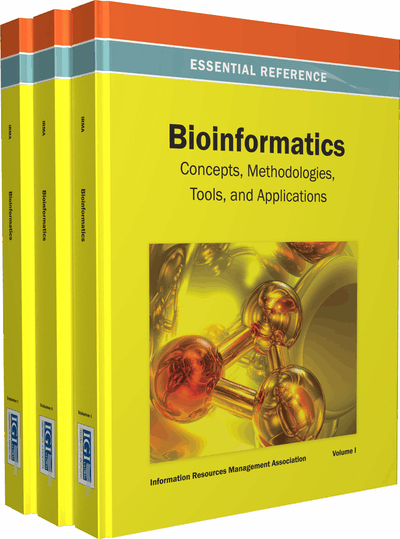# An Optimization to Protein Coding Regions Identification in Eukaryotes

Muneer Ahmad (King Faisal University, Saudi Arabia), Azween Abdullah (University Technology PATRONAS, Malaysia) and Noor Zaman (King Faisal University, Saudi Arabia)
DOI: 10.4018/978-1-4666-3604-0.ch092
Available
\$37.50
No Current Special Offers

## Abstract

Significant improvement in coding regions identification was observed over many real datasets, which were obtained from the national center for bioinformatics. Quantitatively, the authors monitored a gain of 80.5% in coding identification with the Complex method, 42.5% with the Binary method, and 15% with the EIIP indicator sequence method over Mus Musculus Domesticus (House rat), NCBI Accession number: NC_006914, Length of gene: 7700 bp with number of coding regions: 4. Continuous improvement in significance with dyadic wavelet transforms will be observed as a future expectation.
Chapter Preview
Top

## Introduction

In genetic sequences, exonic and intronic regions are identified by discrimination measure that calculates the degree of significance in the form of distinguished boundaries of genic regions in 1/f noise (Shuo & Yi-Sheng, 2009; Roy, Biswas, & Barman, 2009). Higher value of this measure relates to the peaks heights in power spectral estimation. Period three property greatly helps in identification of exons from introns.

DFT (Akhtar, Ambikairajah, & Epps, 2008; Hota & Srivastava, 2008), STFT (George & Thomas, 2010), convolution, windowing, splicing, and wavelet (Datta & Asif, 2005) transforms provide a foundation for DNA signal processing, denoising and optimal framework provision towards the accurate prediction of genic regions in intron-exon mix molecules.

The transformation of a complex valued function into another complex valued function (Hota & Srivastava, 2008) defined over a real variable or simply the transformation of time domain function / signal to a frequency domain function / signal. Fourier transform is normally used to visualize the frequency components of a signal. It helps in better understanding of a time domain signal as timed information at many instances may provide information into the nature, behavior and function of signal; it can be better approximated using frequency domain analysis.

where x (t) is a continuous signal sampled over discrete time intervals (nucleotide samples in a specified gene) and X (f) is a vector representing the frequency components of DNA signal.

The above expression is the Discrete Fourier Transform of DNA signals (Akhtar, Epps, & Ambikairajah, 2008), xn is a DNA signal sampled over N points and exponential e serves as cube root of unity and also provides sinusoidal components of signal. Xk stores the coefficients of this transformation which later can be used for frequency, magnitude and power depiction of signal.

Another important expression / transform for DNA signal analysis is Short Time Fourier Transform STFT which involves the concept of windowing the DFT of a signal.

The gene data is expressed in the form of nucleotides A, T, G, C (Hamdani & Shukri, 2008; Kakumani, Devabhaktuni, & Ahmad, 2008; Mena-Chalco, Carrer, Zana, & Cesar, 2008). Binary indicator sequence method help us in translation of this data into numeric format that later can be used for spectral analysis of DNA signal. This method prices 1 and 0 for the existence or non existence of a specific nucleotide in strand.

In EIIP method, one indicator sequence is proposed as against four binary indicator sequences which computationally reduce the overhead by 75%.

YEIIP = WAXA + WTXT + WCXC + WGXG

Where numerical values are:

• A = 0.1260

• T = 0. 1335

• G= 0. 0806

• C = 0.1340

As a replacement of binary indicator sequence, complex indicator sequence uses one sequence of values namely:

• X (A) = +1

• X (T) = +j

• X (G) = -1

• X (C) = -j

The method of Complex Indicator Sequence reduces the computational overhead by 75% and provides more accurate prediction of genic regions.

In UTP (University Technology PETRONAS) indicator sequence, Nucleotides A, T, G, and C have values Adenine (A) = 0.260, Thymine (T) = 0.375, Guanine (G) = 0.125 and Cytosine (C) = 0.370 using UTP indicator sequence (most significant for exon prediction).

## Complete Chapter List

Search this Book:
Reset